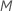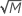# Methods for Random Forests® Regression

###### Note

A Random Forests® model is an approach to solving classification and regression problems. The approach is both more accurate and more robust to changes in predictor variables than a single classification or regression tree. A broad, general description of the process is that Minitab Statistical Software builds a single tree from a bootstrap sample. Minitab randomly selects a smaller number of predictors out of the total number of predictors to evaluate the best splitter at each node. Minitab repeats this process to grow many trees. In the regression case, the prediction from the model is the average of the predictions from all of the individual trees.

To build a regression tree, the algorithm uses the least squares criterion to measure the impurity of nodes. For more details on the construction of a regression tree, go to Node splitting methods in CART® Regression. Details that are specific to Random Forests® follow.

## Bootstrap samples

To build each tree, the algorithm selects a random sample with replacement (bootstrap sample) from the full data set. Usually, each bootstrap sample is different and can contain a different number of unique rows from the original data set. If you use only out-of-bag validation, then the default size of the bootstrap sample is the size of the original data set. If you divide the sample into a training set and a test set, then the default size of the bootstrap sample is the same as the size of the training set. In either case, you have the option to specify that the bootstrap sample is smaller than the default size. On average, a bootstrap sample contains about 2/3 of the rows of data. The unique rows of data that are not in the bootstrap sample are the out-of-bag data for validation.

## Random selection of predictors

At each node in the tree, the algorithm randomly selects a subset of the total number of predictors,, to evaluate as splitters. By default, the algorithm choosespredictors to evaluate at each node. You have the option to choose a different number of predictors to evaluate, from 1 to. If you choosepredictors, then the algorithm evaluates every predictor at every node, resulting in an analysis with the name "bootstrap forest."

In an analysis that uses a subset of predictors at each node, the evaluated predictors are usually different at each node. The evaluation of different predictors makes the trees in the forest less correlated with each other. The less-correlated trees create a slow learning effect so that the predictions improve as you build more trees.

## Validation with out-of-bag data

The unique rows of data that are not part of the tree-building process for a given tree are the out-of-bag data. Calculations for measures of model performance use the out-of-bag data. For more details, go to Methods and formulas for the model summary in Random Forests® Regression.

For a given tree in the forest, a prediction for a row in the out-of-bag data is made from the single tree. The prediction for a row in out-of-bag data is the average of the predictions from the individual trees.

## Prediction for a row in the training set

Each tree in the forest makes an individual prediction for every row in the training set. The predicted value for a row in the training set is the average of the predicted values from all the trees in the forest.

By using this site you agree to the use of cookies for analytics and personalized content.  Read our policy